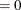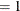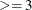### OUTSTAT= Data Set

PROC ARIMA writes the diagnostic statistics for a model to an output data set when the OUTSTAT= option is specified in the ESTIMATE statement. The OUTSTAT data set contains the following:

• the BY variables.

• _MODLABEL_, a character variable that contains the model label, if it is provided by using the label option in the ESTIMATE statement (otherwise this variable is not created).

• _TYPE_, a character variable that contains the estimation method used. _TYPE_ can have the value CLS, ULS, or ML.

• _STAT_, a character variable that contains the name of the statistic given by the _VALUE_ variable in this observation. _STAT_ takes on the values AIC, SBC, LOGLIK, SSE, NUMRESID, NPARMS, NDIFS, ERRORVAR, MU, CONV, and NITER.

• _VALUE_, a numeric variable that contains the value of the statistic named by the _STAT_ variable.

The observations contained in the OUTSTAT= data set are identified by the _STAT_ variable. A description of the values of the _STAT_ variable follows:

AIC

Akaike’s information criterion

SBC

Schwarz’s Bayesian criterion

LOGLIK

the log-likelihood, if METHOD=ML or METHOD=ULS is specified

SSE

the sum of the squared residuals

NUMRESID

the number of residuals

NPARMS

the number of parameters in the model

NDIFS

the sum of the differencing lags employed for the response variable

ERRORVAR

the estimate of the innovation variance

MU

the estimate of the mean term

CONV

tells if the estimation converged. The value of 0 signifies that estimation converged. Nonzero values reflect convergence problems.

NITER

the number of iterations

Remark. CONV takes an integer value that corresponds to the error condition of the parameter estimation process. The value of 0 signifies that estimation process has converged. The higher values signify convergence problems of increasing severity. Specifically:

• CONVindicates that the estimation process has converged.

• CONVorindicates that the estimation process has run into numerical problems (such as encountering an unstable model or a ridge) during the iterations.

• CONVindicates that the estimation process has failed to converge.